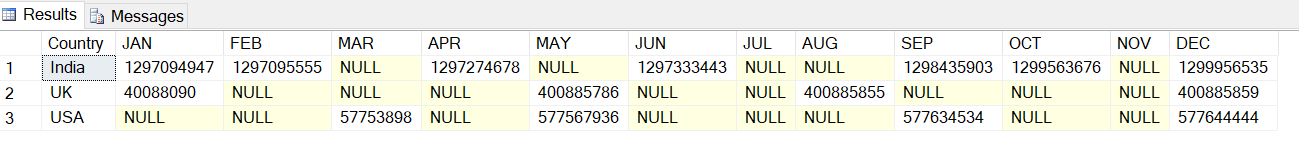In this example we will see how to use PIVOT in SQL. If you have new to PIVOT then you must learn what is PIVOT and what's benefit of using that.

What is PIVOT in SQL ?
Pivot is a SQL server operator that can transform unique values from one column in a result-set into multiple columns in the output , hence it seems like rotating the table OR simply we can remembered that PIVOT is very useful technique to transform or display data Row level to Column.

Example
Suppose we have a Population table of the multiple countries of 2019, the population count did in several months of the year. We want the output that Population count of country month wise.

We have a population table with below information.
```CREATE TABLE [dbo].[T_Population](
[Id] [int] IDENTITY(1,1) NOT NULL,
[Country] [varchar](50) NULL,
[PopulationCountDate] [date] NULL,
[PopulationCount] [int] NULL,
CONSTRAINT [PK_T_Population] PRIMARY KEY CLUSTERED
(
[Id] ASC
)WITH (PAD_INDEX = OFF, STATISTICS_NORECOMPUTE = OFF, IGNORE_DUP_KEY = OFF,
ALLOW_ROW_LOCKS = ON, ALLOW_PAGE_LOCKS = ON)
ON [PRIMARY]
) ON [PRIMARY]
GO
GO  ```
Using the below PIVOT script we can make the row into column.
```SELECT *
FROM (
SELECT Country,
left(datename(month,PopulationCountDate),3)as [month], PopulationCount
FROM T_Population
) as s
PIVOT
(
SUM(PopulationCount) FOR [month] IN (JAN, FEB, MAR, APR,MAY, JUN,
JUL, AUG, SEP, OCT, NOV, DEC)
)AS pvt  ```The Output Should like this.
Boom, You can see the SQL rows converted into columns.

Summary
In this tutorial we discussed how to use PIVOT in SQL. If have any question related to this topic then give your feedback.